# Cray Reveal¶

## Description¶

Cray Reveal is part of the Cray Perftools software package. It utilizes the Cray CCE program library (hence it only works under PrgEnv-cray) for source code analysis, combined with performance data collected from CrayPat. Reveal helps to identify top time-consuming loops, with compiler feedback on dependency and vectorization.

Attempting to achieve best performance on today's supercomputers demands that besides using MPI between nodes or sockets, the developer must also use a shared-memory programming paradigm on the node and vectorize low-level looping structures.

The process of adding parallelism involves finding top serial work-intensive loops, performing parallel analysis, scoping and vectorization, adding OpenMP layers of parallelism, and analyzing performance for further optimizations, specifically vectorization of innermost loops. Cray Reveal can be used to simplify these tasks. Its loop scope analysis provides variable scope and compiler directive suggestions for inserting OpenMP parallelism to serial or pure MPI code.

## Steps to Use Cray Reveal¶

Reveal is available on Cori under PrgEnv-cray by loading the Cray perftools module. It has conflicts with the NERSC default loaded darshan module (used for collecting I/O information). Reveal has a GUI interface, so it needs X11 access (ssh -XY or NX).

### 1. Basic Steps to Setup User Environment¶

nersc$module swap PrgEnv-intel PrgEnv-cray nersc$ module unload darshan
nersc$module load perftools-base nersc$ module load perftools


Note

Once the perftools-base module is loaded, the perftools version will be matched to the corresponding perftools-base module.

### 2. Generate Loop Work Estimates¶

#### a. Build with -h profile_generate¶

Fortran code example:

nersc$ftn -c -h profile_generate myprogram.f90 nersc$ ftn -o myprogram -h profile_generate myprogram.o


C code example (this code will be used in following steps):

nersc$cc -c -h profile_generate myprogram.c nersc$ cc -o myprogram -h profile_generate myprogram.o


Note

It is a good idea to separate compilation and linking to preserve object files. It is also suggested to separate this step from generating the program library (with -hpl) since -h profile_generate disables all optimizations.

nersc$pat_build -w myprogram  The executable myprogram+pat will be generated. Here, the -w flag is used to enable tracing. #### c. Run the Program to Generate Raw Performance Data in *.xf Format¶ Below is a simple batch interactive session example. A regular batch script can also be used to launch the myprogram+pat program. It is recommended that you execute the code from a Lustre file system. nersc$ salloc -q debug -t 30:00 -C knl


Then, use srun to run the code. In this case, 4 MPI tasks are used.

nersc$srun -n 4 ./myprogram+pat  This generates one or more raw data files in *.xf format. Before proceeding, relinquish the job allocation: nersc$ exit


nersc$pat_report myprogram+pat+......xf > myprogram+pat.rpt  ### 3. Generate a Program Library¶ nersc$ cc -O3 -hpl=myprogram.pl -c myprogram.c


Warning

If there are multiple source code directories, this program library directory needs to be an absolute path.

Note

myprograml.pl is a directory; users need to clean it from time to time.

nersc$cp myprogram.c myprogram_orig.c  The Reveal suggested code may overwrite your original version. ### 5. Launch Reveal¶ nersc$ reveal myprogram.pl myprogram+pat+....ap2


(Use the exact *.ap2 file name in the above command.)

### 6. Perform Loop Scoping¶

Choose the "Loop Performance" view from the "Navigation" drop-down list, pick some of the high time-consuming loops, start scoping, and insert directives.

The left-side panel lists the top time consuming loops. The top right panel displays the source code. The right bottom panel displays the compiler information about a loop.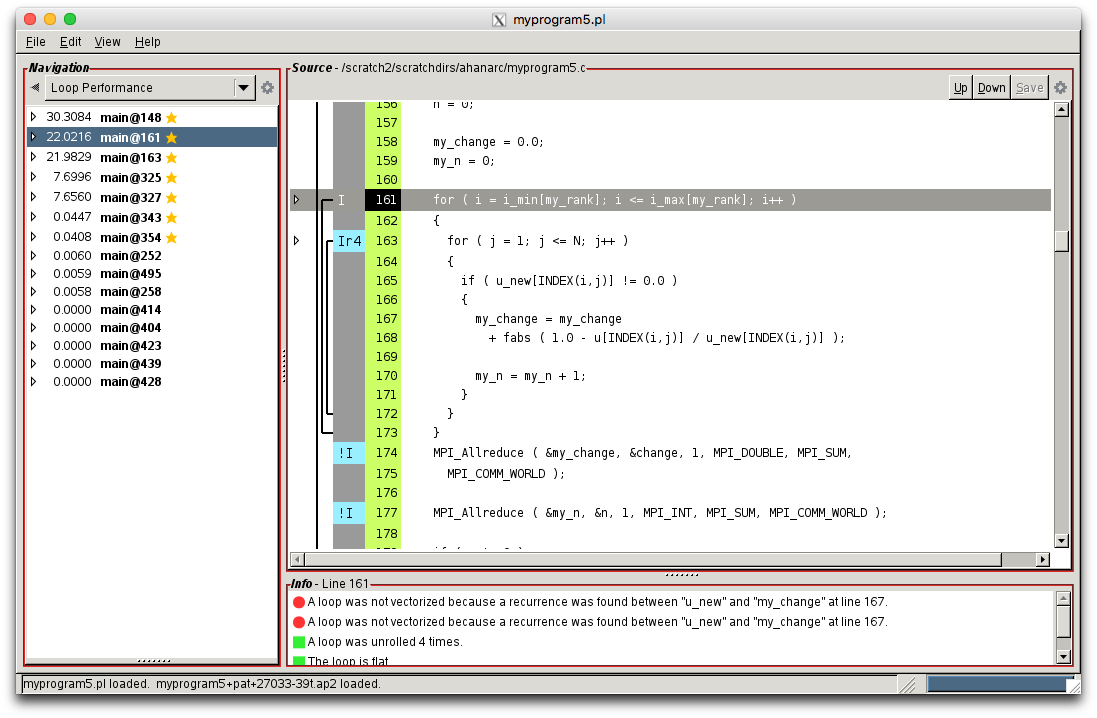Double click a line in the "Info" section to display more explanations of a compiler decision about each loop, such as whether it is vectorized or unrolled.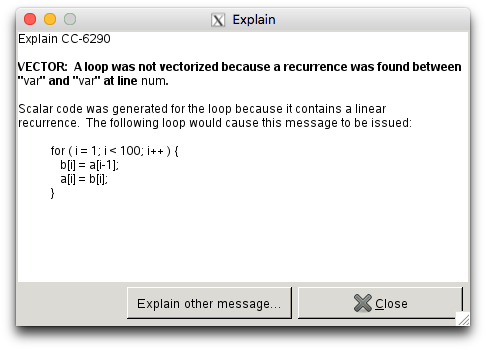Double click a line corresponding to a loop from the section that displays the code and a new "Reveal OpenMP Scoping" window will pop up: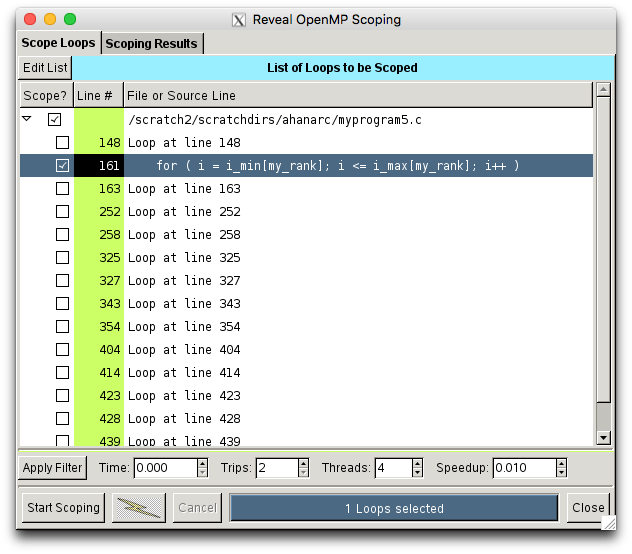Ensure that the only the required loop is checked. Click the "Start Scoping" button on the bottom left. The scoping results for each variable will be provided in the "Scoping Results" tab. Some of the variables are marked red as "Unresolved". The reason why it fails in scoping is also specified.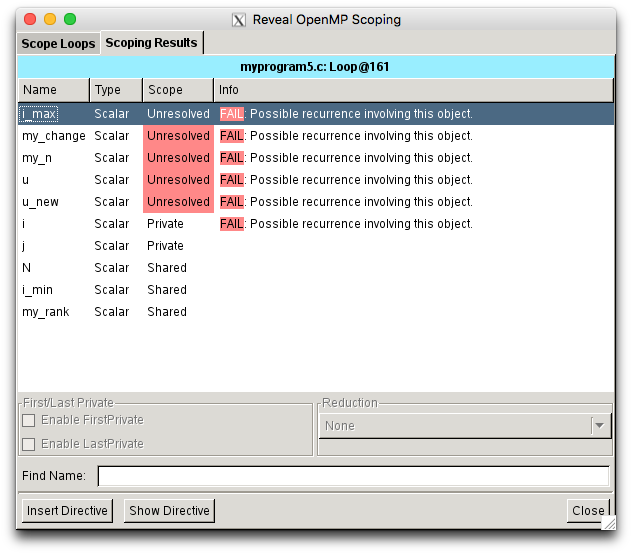Click the "Show Directive" button and the Reveal-suggested OpenMP Directive will be displayed: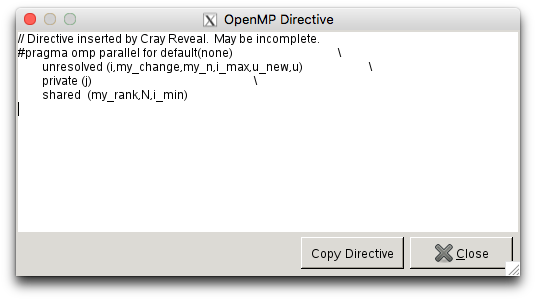Click the "Insert Directive" button to insert the suggested directive in the code: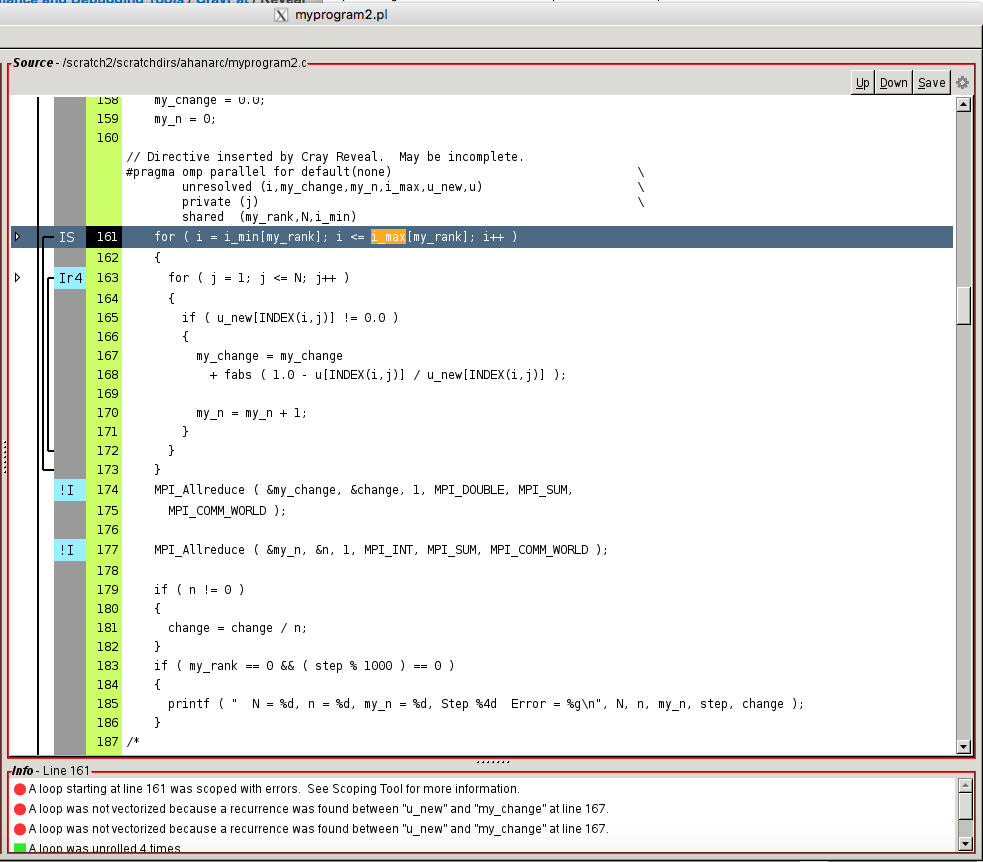Click the "Save" button on the top right hand corner of the main window. A "Save Source" window will pop up: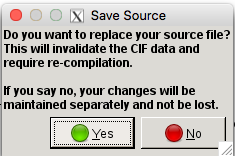Choose "Yes", and a file having the same name as the original file and with the OpenMP directives inserted will be created. The original file will be overwritten.

The above steps can be repeated for one loop at a time. Note that the newly saved file will have the same file name as your original code.

nersc$cp myprogram.c myprogram.c.reveal # (myprogram.c.reveal is the code with OpenMP directives generated by Reveal) nersc$ cp myprogram.c.orig myprogram.c # (these are copies of your original code)
nersc\$ cp myprogram.c.reveal myprogram_omp.c # (myprogram_omp.c is the copy where all the variables will be resolved)


### 7. Work with myprogram_omp.c¶

#### a. Start to resolve all unresolved variables by changing them to private, shared or reduction¶

For example, Reveal provides the following directive for the selected loop:

// Directive inserted by Cray Reveal.  May be incomplete.
#pragma omp parallel for default(none)                      \
unresolved (i,my_change,my_n,i_max,u_new,u)              \
private (j)                                           \
shared  (my_rank,N,i_min)
for ( i = i_min[my_rank]; i <= i_max[my_rank]; i++ )
{
for ( j = 1; j <= N; j++ )
{
if ( u_new[INDEX(i,j)] != 0.0 )
{
my_change = my_change
+ fabs ( 1.0 - u[INDEX(i,j)] / u_new[INDEX(i,j)] );
my_n = my_n + 1;
}
}
}


Note that the keyword unresolved is used above since Reveal could not resolve the data scope. We need to change these to reduction(+:my_change) and shared(u_new,u) and save a new copy of the code as "myprogram_omp.c".

// Directive inserted by Cray Reveal.  May be incomplete.
#pragma omp parallel for default(none)                \
reduction (+:my_change,my_n)                      \
private (i,j)                                \
shared  (my_rank,N,i_min,i_max,u,u_new)
for ( i = i_min[my_rank]; i <= i_max[my_rank]; i++ )
{
for ( j = 1; j <= N; j++ )
{
if( u_new[INDEX(i,j)] != 0.0 )
{
my_change = my_change
+ fabs ( 1.0 - u[INDEX(i,j)] / u_new[INDEX(i,j)] );
my_n = my_n + 1;
}
}
}


#### b. Compile with OpenMP enabled.¶

This can be done under any PrgEnv. Make sure to resolve compilation warnings and errors.

Note

Use --cpus-per-task=num_threads if you are using srun to execute your code.

### Output for MPI code only (myprogram.c) using 4 MPI Processes¶

POISSON_MPI!!
C version
2-D Poisson equation using Jacobi algorithm
===========================================
MPI version: 1-D domains, non-blocking send/receive
Number of processes         = 4
Number of interior vertices = 1200
Desired fractional accuracy = 0.001000

N = 1200, n = 1373424, my_n = 326781, Step 1000  Error = 0.143433
N = 1200, n = 1439848, my_n = 359992, Step 2000  Error = 0.0442104
N = 1200, n = 1439907, my_n = 359994, Step 3000  Error = 0.0200615
N = 1200, n = 1439928, my_n = 360000, Step 4000  Error = 0.0114007
N = 1200, n = 1439936, my_n = 359983, Step 5000  Error = 0.0073485
N = 1200, n = 1439916, my_n = 359983, Step 6000  Error = 0.00513294
N = 1200, n = 1439935, my_n = 359996, Step 7000  Error = 0.00379038
N = 1200, n = 1439915, my_n = 359997, Step 8000  Error = 0.00291566
N = 1200, n = 1439950, my_n = 359997, Step 9000  Error = 0.00231378
N = 1200, n = 1439982, my_n = 360000, Step 10000  Error = 0.00188195
N = 1200, n = 1439988, my_n = 360000, Step 11000  Error = 0.00156145
N = 1200, n = 1439983, my_n = 360000, Step 12000  Error = 0.00131704
N = 1200, n = 1439983, my_n = 360000, Step 13000  Error = 0.00112634

Wall clock time =29.937182 secs

POISSON_MPI:

Normal end of execution.


### Output for MPI+OpenMP code (myprogram_omp.c) using 4 MPI Processes with 4 OpenMP Threads per MPI Process¶

POISSON_MPI!!
C version
2-D Poisson equation using Jacobi algorithm
===========================================
MPI version: 1-D domains, non-blocking send/receive
Number of processes         = 4
Number of interior vertices = 1200
Desired fractional accuracy = 0.001000

N = 1200, n = 1373424, my_n = 326781, Step 1000  Error = 0.143433
N = 1200, n = 1439848, my_n = 359992, Step 2000  Error = 0.0442104
N = 1200, n = 1439907, my_n = 359994, Step 3000  Error = 0.0200615
N = 1200, n = 1439928, my_n = 360000, Step 4000  Error = 0.0114007
N = 1200, n = 1439936, my_n = 359983, Step 5000  Error = 0.00734855
N = 1200, n = 1439916, my_n = 359983, Step 6000  Error = 0.00513294
N = 1200, n = 1439935, my_n = 359996, Step 7000  Error = 0.00379038
N = 1200, n = 1439915, my_n = 359997, Step 8000  Error = 0.00291566
N = 1200, n = 1439950, my_n = 359997, Step 9000  Error = 0.00231378
N = 1200, n = 1439982, my_n = 360000, Step 10000  Error = 0.00188195
N = 1200, n = 1439988, my_n = 360000, Step 11000  Error = 0.00156145
N = 1200, n = 1439983, my_n = 360000, Step 12000  Error = 0.00131704
N = 1200, n = 1439983, my_n = 360000, Step 13000  Error = 0.00112634

Wall clock time = 12.807604 secs
POISSON_MPI:

Normal end of execution.


### Results¶

The following results were obtained by executing on Edison for 4 MPI tasks.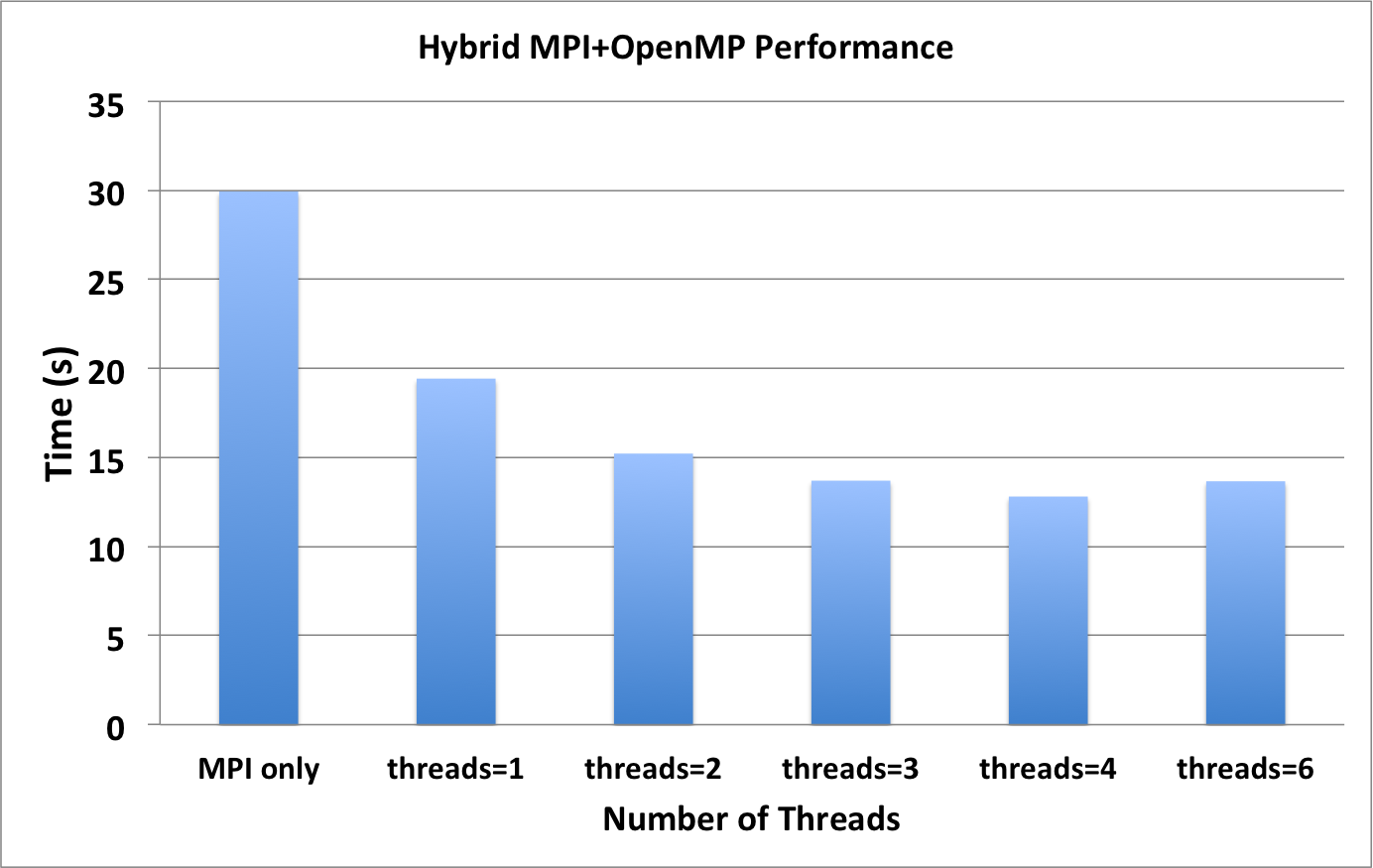## Issues and Limitations¶

• Cray Reveal works only under PrgEnv-cray with the CCE compiler
• There will be unresolved and incomplete variable scopes
• There may be more incomplete and incorrect variables identified when compiling OpenMP code
• The user still needs to understand OpenMP and resolve the issues
• Reveal does not insert OpenMP tasks, barrier, critical, atomic, etc. regions